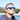# JS Design Patterns - Constructor

Design patterns are reusable solutions that can be applied to common problems in software design. I decided to revisit them with a Javascript approach.

Here I’m starting with the most simple one, the Javascript Constructor Pattern.

There are many different ways to create an object in Javascript, some patterns were created in the early days but with the language evolution, new ways were incorporated to ease the developing process.

## Object Literals

``````const car = {}

car.model = 'Accent'
car.getInfo = function() {
console.log(`Model: \${this.model}`)
}

// or
const car = {
model: 'Accent',
getInfo: function() {
console.log(`Model: \${this.model}`)
},
}

car.getInfo()
// Model: Accent``````

This is the most common way to create an object. Just a simple assignment. It is good for local disposable objects that you have only one instance, usually with only properties and no functions.

When we start to add functions to objects, we are defining pieces of code to be reused, and this becomes a problem when we have to create multiple instances of the same object.

## Factory Function

``````function createCar(model) {
return {
model: model,
getInfo: function() {
console.log(`Model: \${this.model}`)
},
}
}

const car = createCar('Accent')
console.log(car)
// { model: 'Accent', getInfo: [Function: getInfo] }
car.getInfo()
// Model: Accent

const car2 = createCar('Corolla')
car2.getInfo()
// Model: Corolla``````

With this pattern we can create multiple car objects without having to define common behavior many times.

The only problem with this approach is that for every car instance, there is a new instance of the function getInfo, in Javascript functions are objects. If we increase the amount of car instances, it can bloat the memory.

We could fix it like this

``````const Car = {
getInfo: function() {
console.log(`Model: \${this.model}`)
},
}

function createCar(model) {
const _car = Object.create(Car)
_car.model = model
return _car
}

const car = createCar('Accent')
console.log(car)
// { model: 'Accent' }
car.getInfo()
// Model: Accent``````

The car object doesn’t have the function getInfo but when we call it, it outputs the correct message. Why?

## Prototype Chain

In Java, every class extends the super class Object, in Javascript it is almost the same. Every time we instantiate an object in Javascript, it receives the Object.prototype.

``````const obj = {}
Object.getPrototypeOf(obj) === Object.prototype
// true``````

So when we access a property or a function in an object instance, the object is examined for that property, if it doesn’t exist, it’s prototype is examined next and so on. That’s why in the previous section, when we called getInfo we got the message correctly.

The Object.create function instantiate a new object and set an object as the prototype of this new instance.

``````const Car = {
getInfo: function() {
console.log(`Model: \${this.model}`)
},
}

Object.getProtorypeOf(Car) === Object.prototype
// true

const car = Object.create(Car)
Object.getProtorypeOf(car)
// { getInfo: [Function: getInfo] }

Object.getPrototypeOf(car) === Car
// true

Object.getPrototypeOf(car) === Object.prototype
// false``````

This is what’s called the prototype chain `Object <-- Car <-- car`

A better way to create an object is using the new keyword. When we use it in front of a function, we are telling Javascript that the function should behave like a constructor and instantiate a new object. And the instantiated object also receives the constructor’s prototype.

``````function Car(model) {
this.model = model

this.getInfo = function() {
console.log(`Model: \${this.model}`)
}
}

const car = new Car('Accent')

Object.getPrototypeOf(car)
// Car {}``````

But here we are facing the same problem we had with the factory function, every time we instantiate a new Car, the function getInfo gets redefined. But now we can fix the problem setting the function in the function prototype.

``````function Car(model) {
this.model = model
}

Car.prototype.getInfo = function() {
console.log(`Model: \${this.model}`)
}

const car = new Car('Accent')

Object.getPrototypeOf(car)
// Car { getInfo: [Function] }``````

## ES6 classes

In the 6th version of Javascript, the support for classes was added and now we can create objects like in the classical languages.

``````class Car {
constructor(model) {
this.model = model
}

getInfo() {
console.log(`Model: \${this.model}`)
}
}

const car = new Car('Accent')``````

Don’t get fooled, though. Under the hood, this is just a pretty way of defining a constructor function and adding the methods to the prototype chain.

## Assigning and Accessing Properties

``````const car = {}

// Dot syntax
obj.model = 'Accent'
let model = obj.model

// Square brackets syntax
obj['model'] = 'Accent'
let model = obj['model']

// Object.defineProperty
Object.defineProperty(car, 'model', {
value: 'Accent',
writable: true,
enumerable: true,
configurable: true,
})

// Object.defineProperties
Object.defineProperties(car, {
model: {
value: 'Accent',
writable: true,
enumerable: true,
},

getInfo: {
value: function() {
console.log(`Model: \${this.model}`)
},
writable: false,
},
})``````

If you need to create simple disposable objects, use the object literal `{}`, that’s the easiest way, but if you need to add functionality to it, go with the ES6 class syntax, it’s clean, readable, efficient and it’s the official way of doing it. If you need to support old browsers like IE9, you’ll probably need a transpiler like Babel but that’s a subject for another post.

That’s it folks!